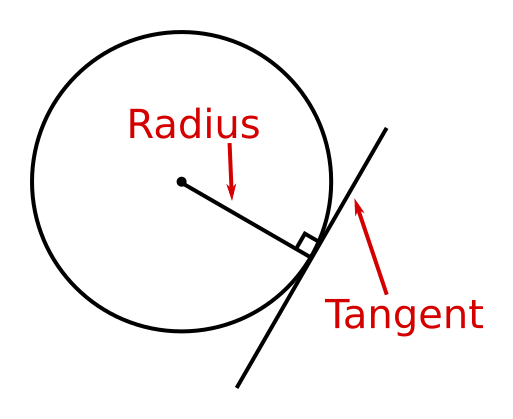# Tangent and radius of a circle meet at 90°

Martin McBride, 2020-08-16
Categories maths gcse geometry

A tangent is a line that just touches the circle at a single point on its circumference.If we draw a radius that meets the circumference at the same point, the angle between the radius and the tangent will always be exactly 90°.

This theorem is covered in this video on circle theorems:

## Proof

You aren't required to learn this proof for GCSE, it is just here for information.

We want to prove that the angle between the radius AB and the tangent CD is a right angle.

The way we will do this is to take some other point P on the tangent and prove that the line AP cannot be perpendicular CD. If we prove that this cannot be true for any point P, then it follows that AB must be perpendicular to CD.We will start by assuming that ∠APB is a right angle. We will then show that this leads to an impossibility and so cannot be true.

Consider the triangle formed by points A, B and P. Suppose the angle of the triangle at P (that is∠APB) was a right angle. This would mean that the line AB would be the hypotenuse of the triangle.

We know that the hypotenuse of a triangle is always longer than the other two sides, which means that AB must be longer than AP. But that cannot be true. The line AE is the same length as the line AB, because each one is a radius of the circle. AP is clearly longer than AE, therefore AB cannot be longer than AP. So, the angle ∠APB cannot be a right angle.

Since the line AP cannot be perpendicular to the tangent for any P, it follows that AB must be perpendicular to the tangent.